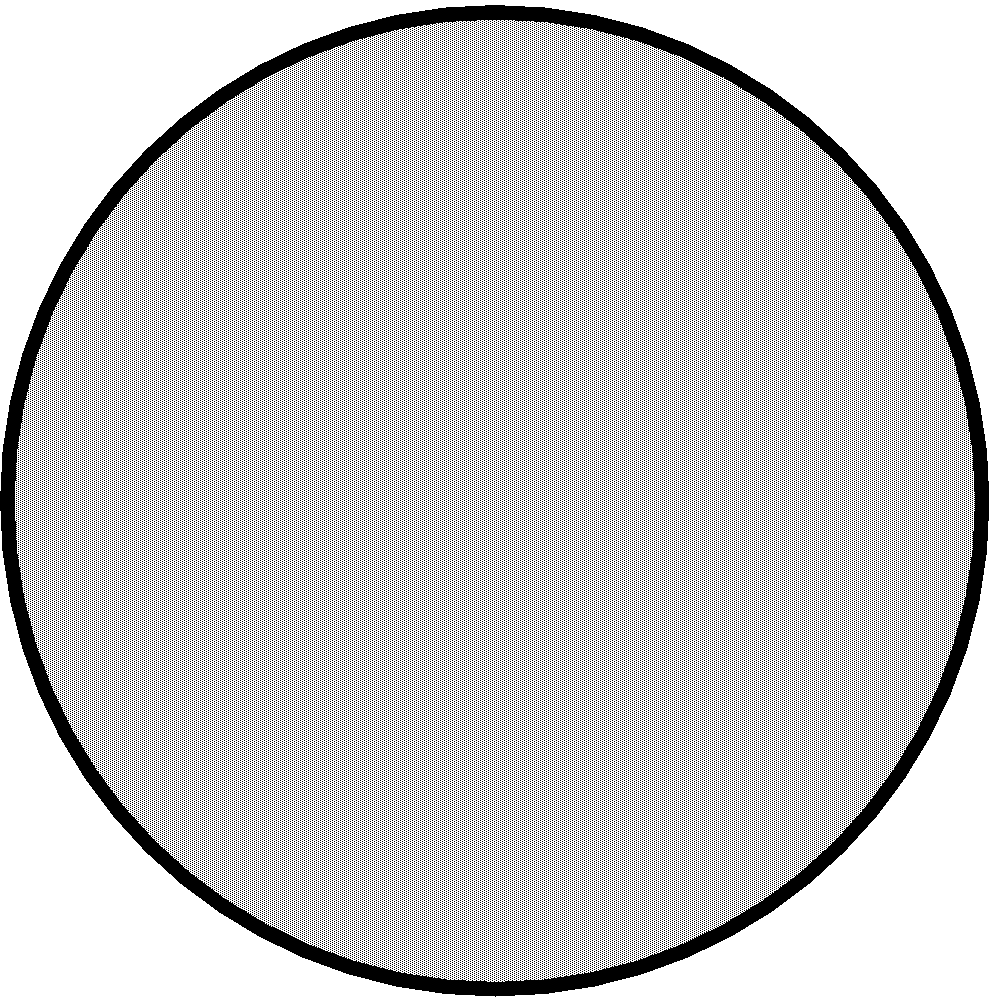# Formula for a sphere

## SPHERE

Objects like a football, a cricket ball, etc., are said to have the shape of a sphere.

FORMULAE For a sphere of radius r, we have

• Volume of spherecubic units

• Surface area of the sphere =sq unitsHEMISPHERE A plane through the centre of a sphere cuts it into two equal parts. Each part is called a hemisphere.

FORMULAE For a hemisphere of radius r, we have

• Volume of the hemispherecubic units
• Curved surface area of the hemisphere = (2πr2) sq units
• Total surface area of the hemisphere = (3πr2) sq units

### SOLVED EXAMPLES

question A sphere of radius 5 cm. find its volume and surface area

Sol.   Here, r = 5cm

So, volume of Sphere iscubic units

== 523.80 cm3

Surface area of sphere is =sq units

= 4×3.14×5×5

= 62.8 cm2

question How many spherical bullets can be made out of a solid cube of lead whose edge measures 44 cm, each bullet being 4 cm in diameter?

Sol. Length of each edge of the cube = 44 cm

Volume of the cube = (44 × 44 × 44) cm3

Radius of each bullet, r = 2 cm

Volume of each bulletNumber of bullets formedHence, the number of bullets formed is 2541.

## CBSE NCERT Solutions for Class 8 Maths

Notes,worksheet and solved question for Maths class 8

Check your marks in a chapter which you have complited in school from Physics Wallah chapter wise online test just click on the link given below